# Basic Geometry : How to find circumference

## Example Questions

← Previous 1 3 4 5 6 7 8 9 10 11 12

### Example Question #1 : How To Find Circumference

If this circle has a diameter of 12 inches, what is its circumference?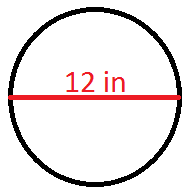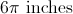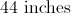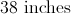none of these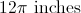Explanation:

Know that the formula for circumference is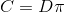, where C is the circumference and D is the diameter.  It is given that the diameter is 12 inches.  Therefore, the circumference is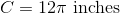### Example Question #1 : How To Find Circumference

A circle is circumscribed around a square that has sides of 5cm. What is the perimeter of the circle?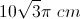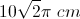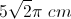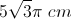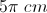Explanation:

Because the circle is circumscribed around the square, the diagonal of the square is the same as the diameter of the circle. So to find the diagonal we use the Pythagorean Theorem: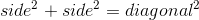So the equation to solve becomes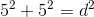or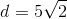The perimeter, or circumference, of the circle is given by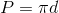or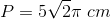### Example Question #3 : How To Find Circumference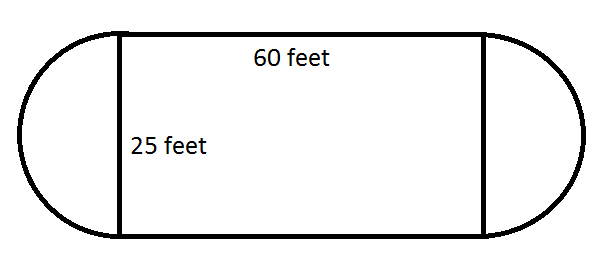Find the perimeter of the ice rink formed by the rectangle and semi-circles with the dimensions shown.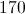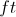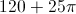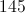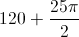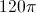Explanation:

The perimeter of the rink runs along the outside of the combined shapes. The two outer edges of the rectangle are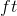each, soof the perimeter consists of those two sides.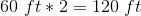The two semi-circles that form the remainder of the perimeter, and can be considered two halves of one circle. The diameter of the circle would be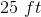. The circumference of the circle would account for the outer edges of the two semi-circles in the ice rink. Find the circumference of the circle with the formula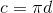as follows.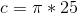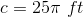Finally, add this circumference that accounts for the semi-circles to the length of the two sides formed by the rectangle to find the total perimeter of the rink.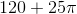### Example Question #1 : How To Find Circumference

If a circle has an area of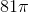, what is the circumference of the circle?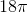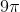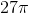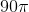Explanation:

The formula for  the area of a circle is πr2. For this particular circle, the area is 81π, so 81π = πr2. Divide both sides by π and we are left with r2=81. Take the square root of both sides to find r=9. The formula for the circumference of the circle is 2πr = 2π(9) = 18π. The correct answer is 18π.

### Example Question #43 : Plane Geometry

A circle with an area of 13π in2 is centered at point C. What is the circumference of this circle?

26π

√26π

√13π

13π

2√13π

2√13π

Explanation:

The formula for the area of a circle is πr2.

We are given the area, and by substitution we know that 13π πr2.

We divide out the π and are left with 13 = r2.

We take the square root of r to find that r = √13.

We find the circumference of the circle with the formula = 2πr.

We then plug in our values to find = 2√13π.

### Example Question #44 : Plane Geometry

A 6 by 8 rectangle is inscribed in a circle. What is the circumference of the circle?

8π

10π

25π

12π

6π

10π

Explanation:

First you must draw the diagram. The diagonal of the rectangle is also the diameter of the circle. The diagonal is the hypotenuse of a multiple of 2 of a 3,4,5 triangle, and therefore is 10.
Circumference = π * diameter = 10π.

### Example Question #1 : How To Find Circumference

A gardener wants to build a fence around their garden shown below. How many feet of fencing will they need, if the length of the rectangular side is 12 and the width is 8?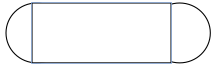96 ft

40 ft.

4π + 24

8π + 24

8π + 24

Explanation:

The shape of the garden consists of a rectangle and two semi-circles. Since they are building a fence we need to find the perimeter. The perimeter of the length of the rectangle is 24. The perimeter or circumference of the circle can be found using the equation C=2π(r), where r= the radius of the circle. Since we have two semi-circles we can find the circumference of one whole circle with a radius of 4, which would be 8π.

### Example Question #1 : How To Find Circumference

The diameter of a circle is defined by the two points (2,5) and (4,6). What is the circumference of this circle?

π√5

2.5π

π√2.5

π√5

Explanation:

We first must calculate the distance between these two points. Recall that the distance formula is:√((x2 - x1)2 + (y2 - y1)2)

For us, it is therefore: √((4 - 2)2 + (6 - 5)2) = √((2)2 + (1)2) = √(4 + 1) = √5

If d = √5, the circumference of our circle is πd, or π√5.

### Example Question #5 : How To Find Circumference

The diameter of a circle is 16 centimeters.  What is the circumference of the circle?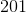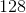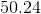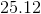Explanation:

To find the circumference of a circle, multiply the circle's diameter by pi (3.14).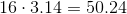### Example Question #6 : How To Find Circumference

A car tire has a radius of 18 inches. When the tire has made 200 revolutions, how far has the car gone in feet?

500π

300π

600π

3600π